• 一、周期为2l的周期函数的傅里叶级数 实际问题中所遇到的周期函数，它的周期不一定是2π2\pi2π。如之前提到的矩形波，它的周期函数是T=2πωT=\frac{2\pi}{\omega}T=ω2π​。因此，这里讨论周期为2l2l2l的周期函数...
一、周期为2l的周期函数的傅里叶级数
实际问题中所遇到的周期函数，它的周期不一定是$2\pi$。如之前提到的矩形波，它的周期函数是$T=\frac{2\pi}{\omega}$。因此，这里讨论周期为$2l$的周期函数的傅里叶级数。根据之前讨论的结果，经过自变量的变量代换，可得到下面的定理：
定理：设周期为2l的周期函数$f(x)$满足收敛定理的条件，则它的傅里叶级数展开式为
$f(x)=\frac{a_0}{2}+\sum_{n=1}^\infty(a_ncos\frac{n\pi x}{l}+b_nsin\frac{n\pi x}{l})(x\in C)$
其中
$a_n=\frac{1}{l}\int_{-l}^lf(x)cos\frac{n\pi x}{l}dx\quad (n=0,1,2,···) \\ b_n=\frac{1}{l}\int_{-l}^l f(x)sin\frac{n\pi x}{l}dx\quad (n=1,2,3,···)\\ C=\{x|f(x)=\frac{1}{2}[f(x^-)+f(x^+)]\}$
当$f(x)$为奇函数时，
$f(x)=\sum_{n=1}^\infty b_nsin\frac{n\pi x}{l}\quad (x\in C)$
其中
$b_n=\frac{2}{l}\int_0^lf(x)sin\frac{n\pi x}{l}dx \quad (n=1,2,3,···)$
当$f(x)$为偶函数时，
$f(x)=\frac{a_0}{2}+\sum_{n=1}^\infty a_ncos\frac{n\pi x}{l}(x\in C)$
其中
$a_n=\frac{2}{l}\int_0^l f(x)cos\frac{n\pi x}{l}dx \quad(n=0,1,2,···)$
二、傅里叶级数的复数形式
傅里叶级数可用复数形式表示，在电子技术中，经常应用这种形式。
设周期为$2l$的周期函数$f(x)$的傅里叶级数为
$\frac{a_0}{2}+\sum_{n=1}^\infty(a_ncos\frac{n\pi x}{l}+b_nsin\frac{n\pi x}{l}) \tag{1}$
其中系数$a_n$与$b_n$为
$a_n=\frac{1}{l}\int_{-l}^lf(x)cos\frac{n\pi x}{l}dx \quad (n=0,1,2,···)\\ b_n=\frac{1}{l}\int_{-l}^lf(x)sin\frac{n\pi x}{l}dx \quad (n=1,2,3,···) \tag{2}$
利用欧拉公式
$cos\,t=\frac{e^{ti}+e^{-ti}}{2},\space sin\,t=\frac{e^{ti}-e^{-ti}}{2i}$
把（1）式化为
$\frac{a_0}{2}+\sum_{n=1}^\infty[\frac{a_n}{2}(e^{\frac{n\pi x}{l}i}+e^{-\frac{n\pi x}{l}i})-\frac{b_ni}{2}(e^{\frac{n\pi x}{l}i}-e^{-\frac{n\pi x}{l}i})] \\ =\frac{a_0}{2}+\sum_{n=1}^\infty[\frac{a_n-b_ni}{2}e^{\frac{n\pi x}{l}i}+\frac{a_n+b_ni}{2}e^{-\frac{n\pi x}{l}i}] \tag{3}$
记
$\frac{a_0}{2}=c_0, \quad \frac{a_n-b_ni}{2}=c_n,\quad \frac{a_n+b_ni}{2}=c_{-n} \quad (n=1,2,3,···) \tag{4}$
则（2）式就表示为
$c_0+\sum_{n=1}^\infty (c_ne^{\frac{n\pi x}{l}i}+c_{-n}e^{-\frac{n\pi x}{l}i})=(c_ne^{\frac{n\pi x}{l}i})_{n=0}+\sum_{n=1}^\infty(c_ne^{\frac{n\pi x}{l}i}+c_{-n}e^{-\frac{n\pi x}{l}i})$
即得傅里叶级数的复数形式为
$\sum_{n=-\infty}^\infty c_ne^{\frac{n\pi x}{l}i}$
为得出系数$c_n$的表达式，把（2）式代入（4），得
$c_0=\frac{a_0}{2}=\frac{1}{2l}\int_{-l}^lf(x)dx ;\\ c_n=\frac{1}{2l}\int_{-l}^l f(x)e^{-\frac{n\pi x}{l}dx} \quad (n=1,2,3, ···) \\ c_{-n}=\frac{a_n+b_ni}{2}=\frac{1}{2l}\int_{-l}^lf(x)e^{\frac{n\pi x}{l}i}dx \quad (n=0, 1, 2,···)$
将已得的结果合并写为
$c_n=\frac{1}{2l}\int_{-l}^lf(x)e^{-\frac{n\pi x}{l}i}dx \quad (n=0,\pm 1,\pm 2, ···)$
这就是傅里叶系数的复数形式。
傅里叶级数的两种形式本质上是一样的，但复数形式比较简洁，且只用一个算式计算系数。


展开全文• 周期函数的傅里叶级数展开周期函数 周期函数 周期函数表达式为： f(x) = f(x + kT) (k = 1,2,3…) 如果该周期函数满足狄利赫里条件，那么该周期可以展开为傅里叶级数： f(t)=a02+∑n=1∞(a0cos⁡(nω1t)+bnsin...
周期函数的傅里叶级数展开周期函数方波信号的傅里叶级数展开
周期函数
周期函数表达式为：
f(x) = f(x + kT)  (k = 1,2,3…)
如果该周期函数满足狄利赫里条件，那么该周期可以展开为傅里叶级数：
$f(t) = \frac{a_{0}}{2} + \sum_{n=1}^\infty(a_{0}\cos{(n\omega_{1}t)}+b_{n}\sin{(n\omega_{1}t)})$
其中傅里叶系数计算如下：
$\frac{a_{0}}{2} = \frac{1}{T}\int_{t_{0}}^{t_{0}+T }{f(t)dt}$
$a_{n} = \frac{2}{T}\int_{t_{0}}^{t_{0}+T}{f(t)\cos{n\omega_{1}tdt}}$
$b_{n} = \frac{2}{T}\int_{t_{0}}^{t_{0}+T}{\sin{n\omega_{1}tdt}}$
方波信号的傅里叶级数展开
常见方波信号有两种，第一种表达式为：
$f(t) = \begin{cases} U &\text{} kT\le t \le (kT+\frac{a}{2}) \\ 0 &\text{}(kT+ \frac{a}{2}) \le t \le (kT + a) \end{cases}$
则方波信号得傅里叶级数展开得系数为：
$\frac{a_{0}}{2} = \frac{U}{2}$
$a_{n} = \frac{U}{n\pi}\sin{n\pi} = 0$
$b_{n} = \frac{2U}{n\pi}$
所以方波函数的傅里叶展开式为：
$f(t) = \frac{U}{2} + \frac{2U}{\pi}\sum_{n=1}^{\infty}{\frac{1}{n} \sin{2\pi f_{1}t}}$
式中：f1 为周期函数的频率。
第二种常见方波表达式为：
$f(t) = \begin{cases} U &\text{} kT\le t \le (kT+\frac{a}{2}) \\ -U &\text{}(kT+ \frac{a}{2}) \le t \le (kT + a) \end{cases}$
则方波信号得傅里叶级数展开得系数为：
$\frac{a_{0}}{2} = 0$
$a_{n} = 0$
$b_{n} = \frac{4U}{n\pi}$
所以方波函数的傅里叶展开式为：
$f(t) = \frac{U}{2} + \frac{4U}{\pi}\sum_{n=1}^{\infty}{\frac{1}{n} \sin{2\pi f_{1}t}}$
式中：f1 为周期函数的频率。


展开全文数据分析 信号处理
• Matlab并没有自带的求解傅里叶级数的函数，本文将介绍如何使用Matlab进周期函数的傅里叶级数分析，内容包括： 1、求解傅里叶级数的系数 2、求N次谐波的叠加函数，画图比较与原函数的差值 3、做出傅里叶级数的幅度...
（一）前言

Matlab并没有自带的求解傅里叶级数的函数，本文将介绍如何使用Matlab进周期函数的傅里叶级数分析，内容包括：

1、求解傅里叶级数的系数

2、求N次谐波的叠加函数，画图比较与原函数的差值

3、做出傅里叶级数的幅度谱与相位谱

（二）傅里叶级数系数的求解

设f(x)为周期为T的周期函数，则我们有傅里叶级数展开式：

根据系数的求解的定义，我们使用int()函数进行积分即可求解，如果f(x)在一个周期内为分段函数的话可能还需分段积分，我是自己写了一个统一处理分段函数积分的函数，当然也可以自己分段写，本质是一样的。这里以一个周期三角函数为例进行求解，三角波函数图像如下：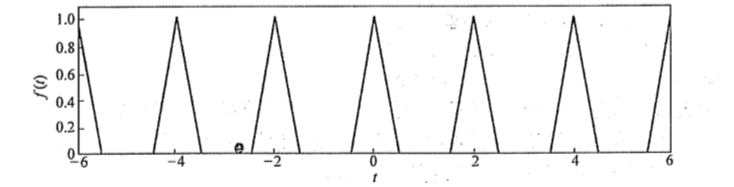则在一个周期内的函数表达式为：

则求解系数的代码如下：

syms t n;
T=2;w=2*pi/T;
f1=2*t+1;f2=-2*t+1;
a0=1/T*myint('t',f1,-0.5,0,f2,0,0.5);
an=myint('t',f1*cos(n*pi*t),-0.5,0,f2*cos(n*pi*t),0,0.5);
bn=myint('t',f1*sin(n*pi*t),-0.5,0,f2*sin(n*pi*t),0,0.5);

运行结果为：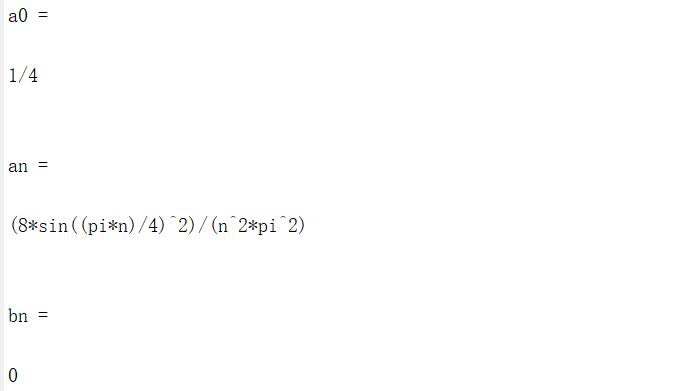（三）作N次谐波的合成图并与原函数进行比较

作合成图实际上就对多个函数进行一定项数的叠加，为了适应不同项数的叠加处理，这里编写函数进行实现：

%trifourierseries.m
function [ f ] = trifourierseries( a0, an, bn, m, t )
%TRIFOURIERSERIES 求傅里叶级数m次谐波的合成
%   a0、an、bn为傅里叶级数的系数
%   t为变量(取样间隔也就是自变量)
f=a0;
syms n;
for n=1:m
f=f+eval(an)*cos(n*pi.*t);
f=f+eval(bn)*sin(n*pi.*t);
end


接着就是进行画图处理：

%求傅里叶变换
t=-6:0.01:6;
d=-6:2:6;
%tripuls为三角波函数，进行偏移叠加处理可以得到一个类似三角周期函数的图
f=pulstran(t,d,'tripuls');
%3次谐波叠加
f3=trifourierseries(a0, an, bn, 3, t);
%9次谐波叠加
f9=trifourierseries(a0, an, bn, 9, t);
%21次谐波叠加
f21=trifourierseries(a0, an, bn, 21, t);
%45次谐波叠加
f45=trifourierseries(a0, an, bn, 45, t);

%级数展开图
subplot(2,3,1);plot(t,f,'r',t,f3,'b');grid on
axis([-6.1,6.1,-0.1,1.1]);title('展开3项');
xlabel('t');ylabel('f(t)');
subplot(2,3,4);plot(t,f,'r',t,f9,'b');grid on
axis([-6.1,6.1,-0.1,1.1]);title('展开9项');
xlabel('t');ylabel('f(t)');
subplot(2,3,2);plot(t,f,'r',t,f21,'b');grid on
axis([-6.1,6.1,-0.1,1.1]);title('展开21项');
xlabel('t');ylabel('f(t)');
subplot(2,3,5);plot(t,f,'r',t,f45,'b');grid on
axis([-6,6,-0.1,1.1]);title('展开45项');
xlabel('t');ylabel('f(t)');

（四）幅度谱与相位图作图

先给定需要绘制的范围，再对具体的幅度An以及相位ψn进行求解，最后画出An~n以及ψn~n即可：

%幅度谱--相位谱
n=0:1:10;
anVal=eval(an);
bnVal=eval(bn);
%注意A0需要自己赋值
An=sqrt(anVal.^2+bnVal.^2);An(1)=a0;
%phi0同理
phi=atan(-bnVal./anVal);phi(1)=0;
subplot(2,3,3);stem(n,An,'b');
grid on; axis([-0.1,10.1,-0.1,1.1]);
title('幅度谱');xlabel('n');ylabel('An');
subplot(2,3,6);plot(n,phi,'b');
grid on; axis([-0.1,10.1,-0.1,1.1]);
title('相位谱');xlabel('n');ylabel('ψn');

（五）最终绘图结果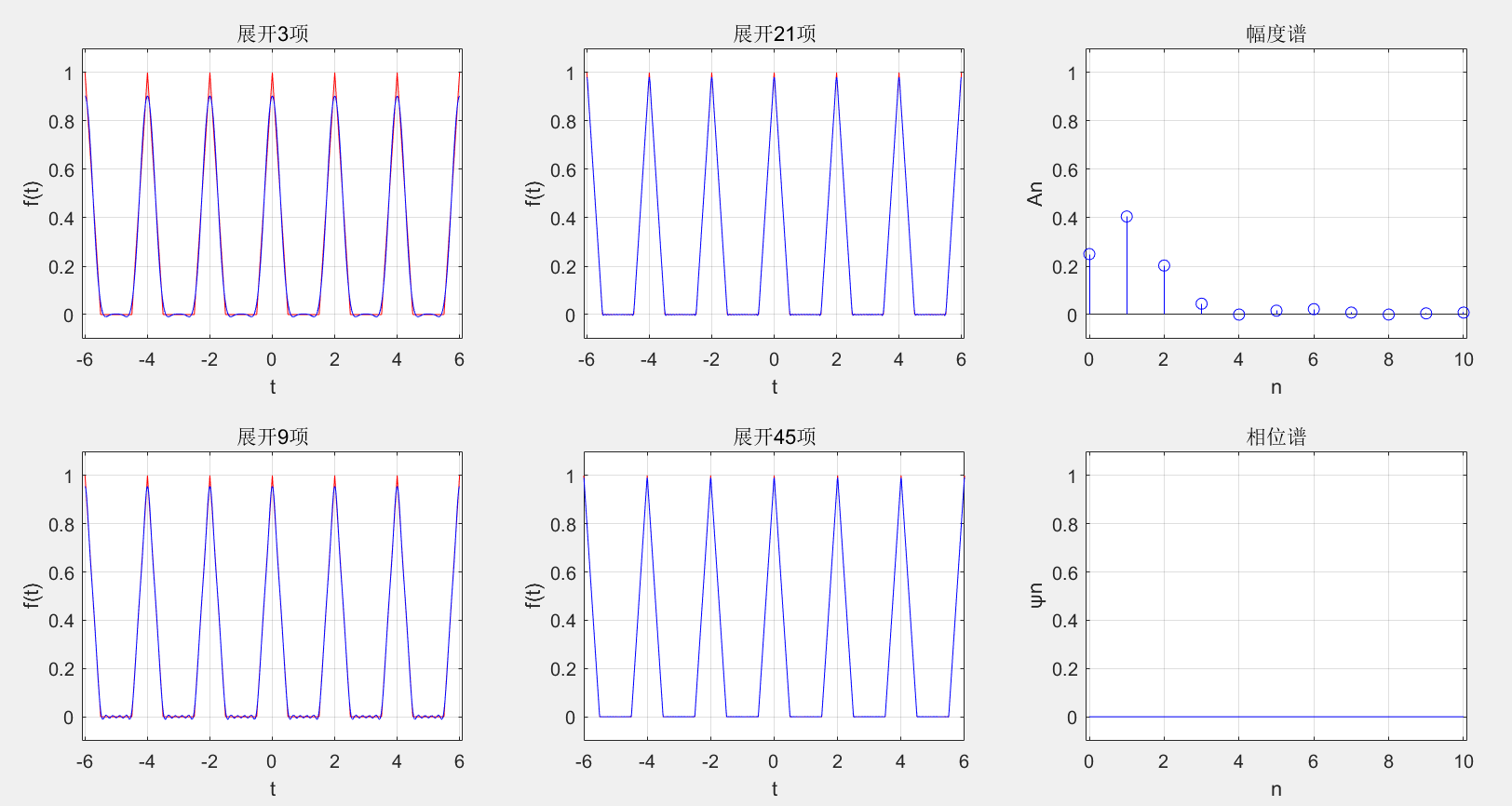（六）说明

该例子为周期三角波函数的求解，如需改成其它函数，只需要将最开始的分段f1、f2以及相应的积分过程进行修改即可。

该程序直接运行的话会比较慢，因为在进行傅里叶级数的计算是将a0、an、bn进行传参然后求解，既涉及到符号运算又涉及到了数值运算，故运算特别慢，在算出a0、an、bn以后，直接将三者的值代入trifourierseries()中即可可以跑得比较快，不过这样的话在修改为不同函数进行积分时，这里也要进行修改，各有所长，也有所缺，看个人所好以及实际情况进行取舍即可。


展开全文Matlab
• 该文件讲解了傅里叶级数定义等和傅里叶级数计算方法等傅立叶级数
• 转载于:https://www.cnblogs.com/tszr/p/11174419.html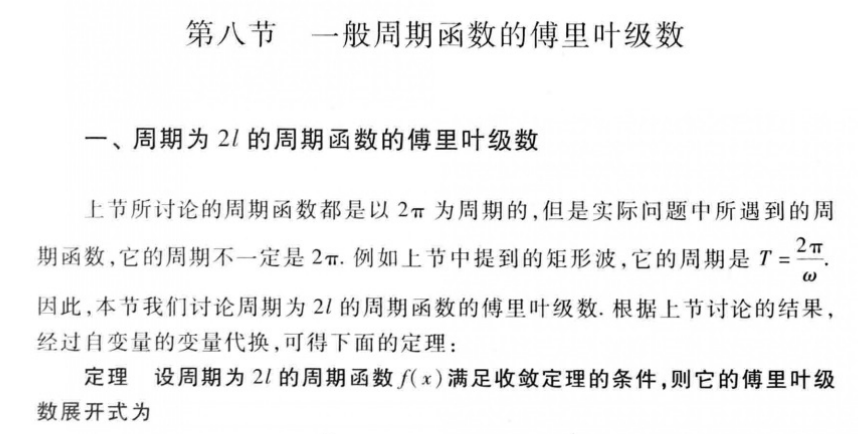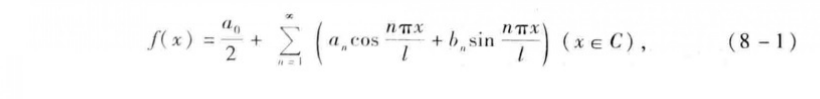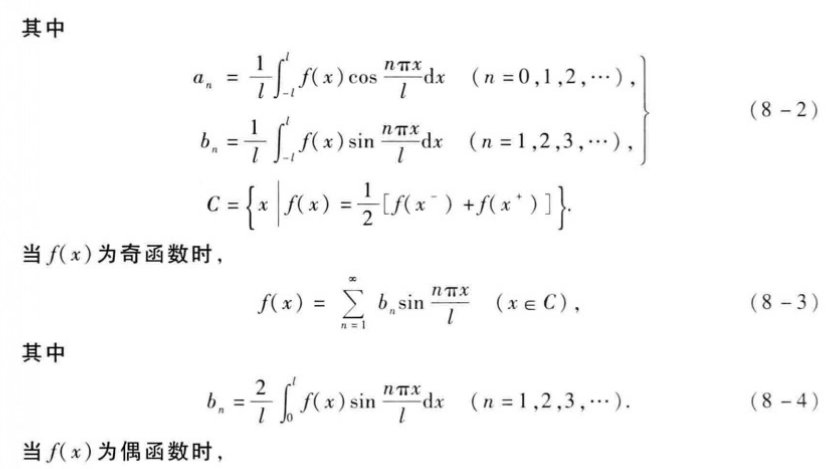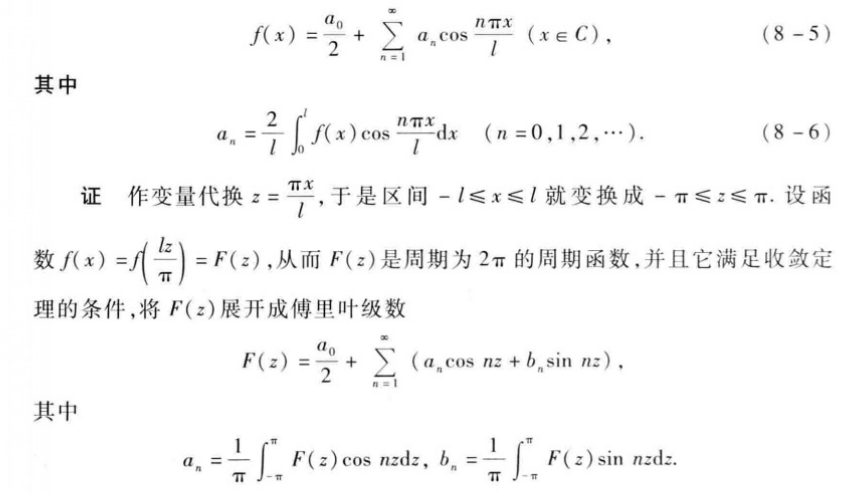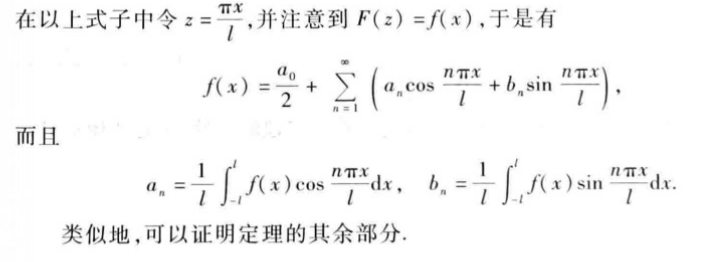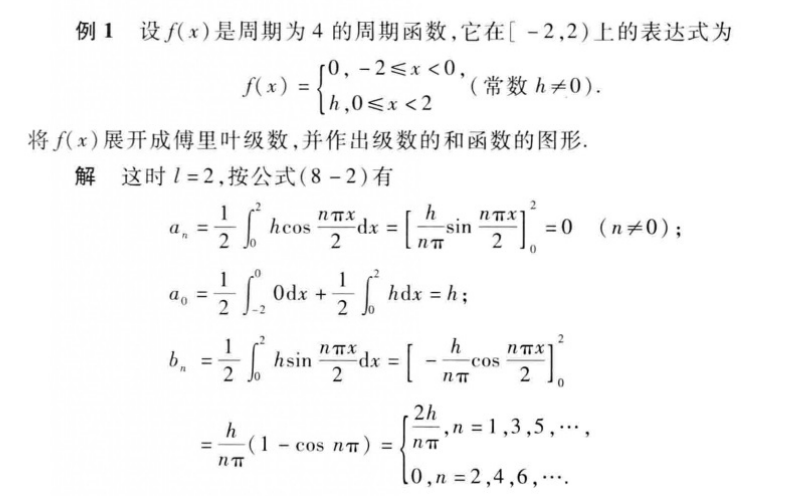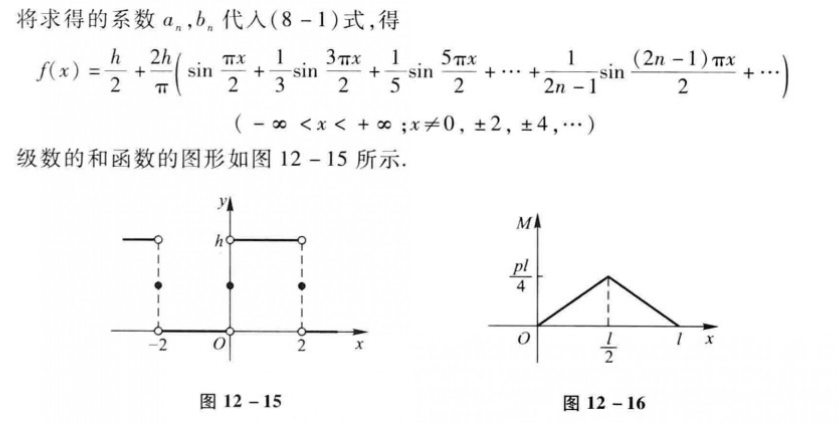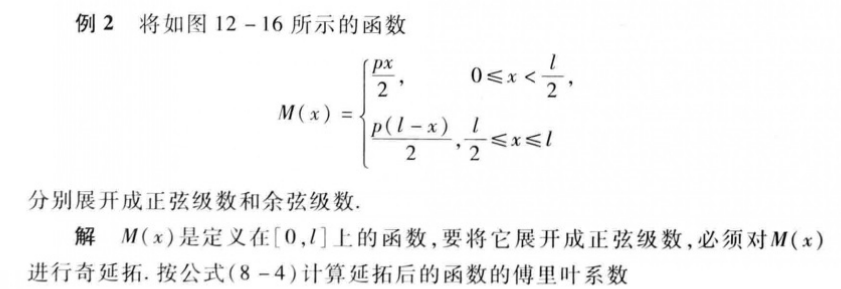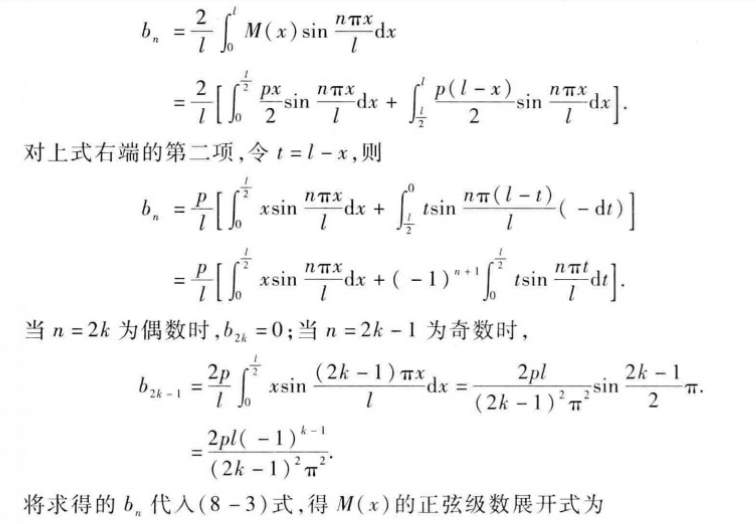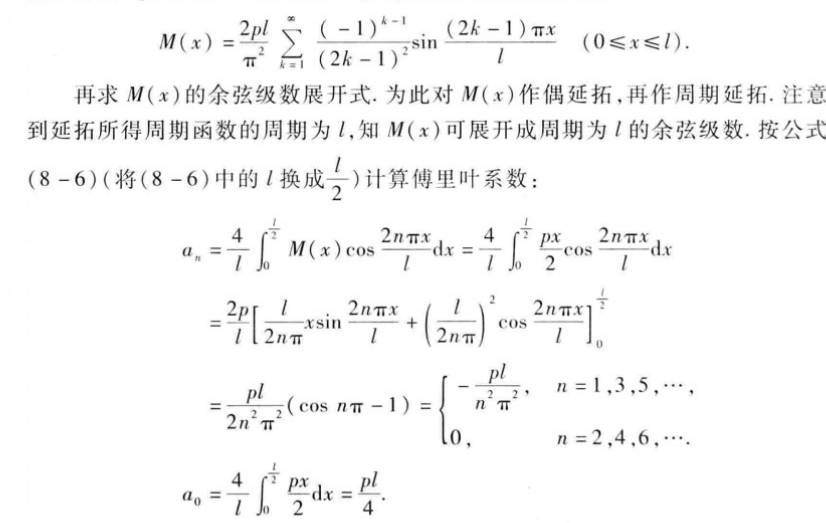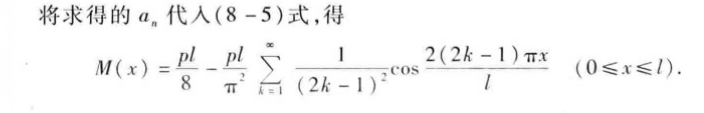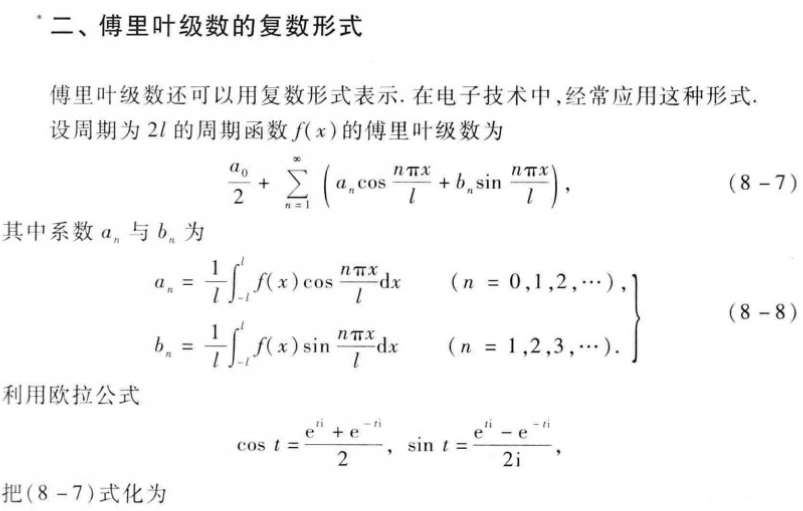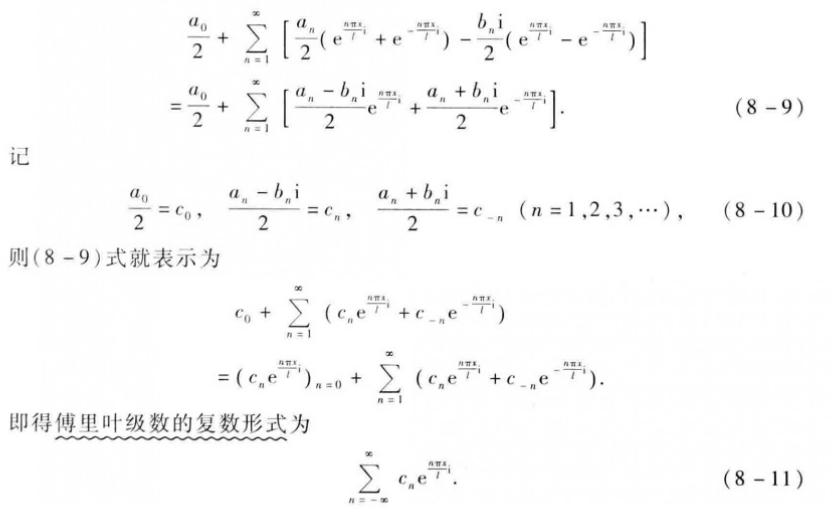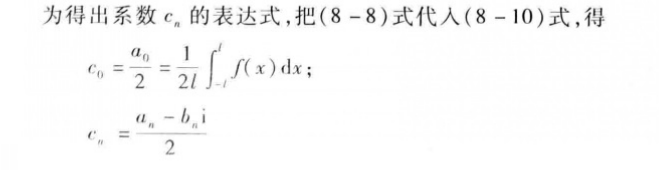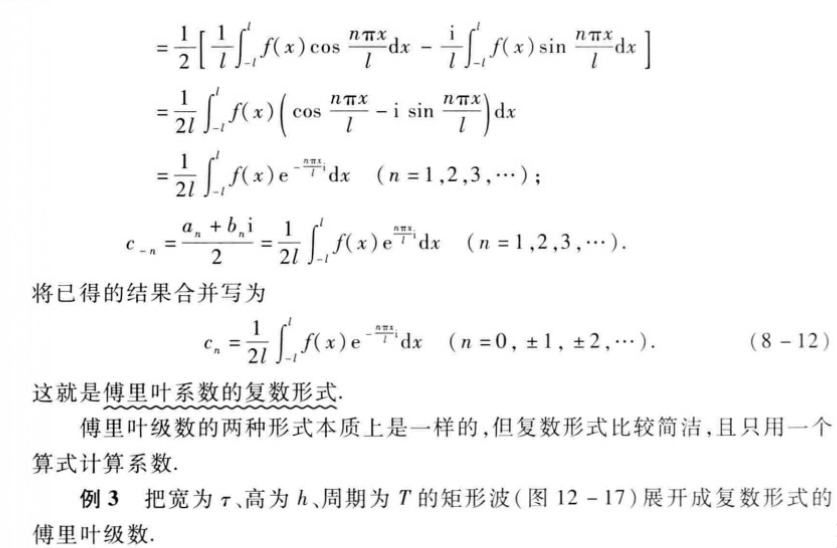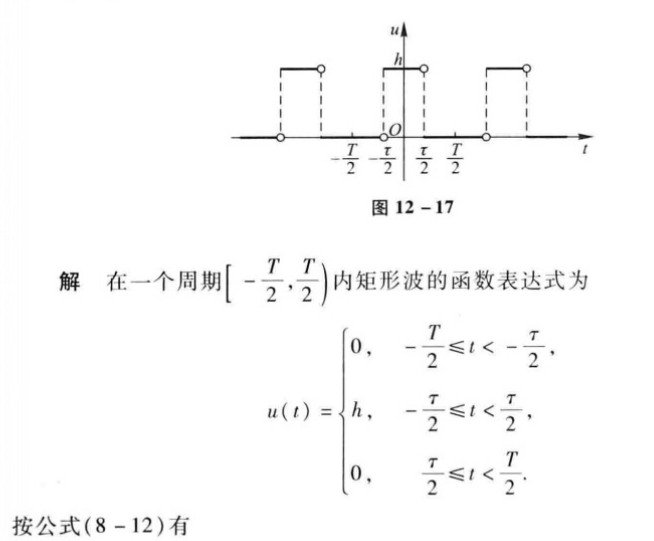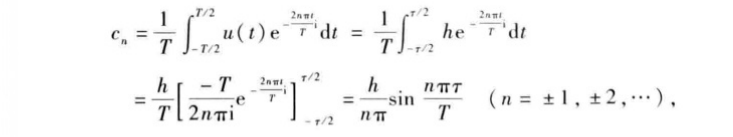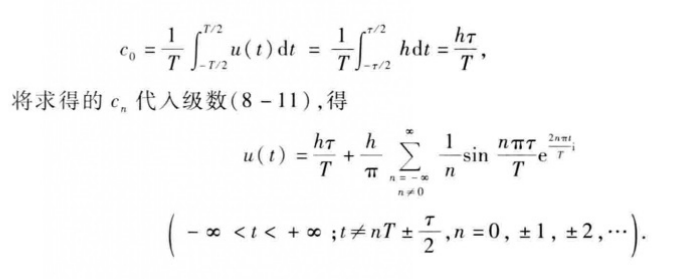转载于:https://www.cnblogs.com/tszr/p/11174419.html
展开全文• 但是，有些函数的傅立叶级数只含有正弦项或只含有余弦项，究其原因，它与所给函数的奇偶性有关。 【定理】以为周期的奇函数展开成傅立叶级数时，它的傅立叶系数适合： 而以为周期的偶函数展开成傅立叶级数时，它...高等数学 无穷级数
• 周期函数傅里叶级数的各次谐波系数确定 在不考虑直流分量的情况下对于周期函数的系数进行计算确定。 简单阐述原理过程。 实例场景： 假定被采样信号的模拟信号时一个周期性时间函数，...周期函数的傅里叶级数为： ...
• W = FOURIERDIFF(V,M,INTERVAL,N) 返回函数的 M 阶导数V 在 INTERVAL 上的 N 个（偶数）等距点采样。 W = FOURIERDIFF(V,M,INTERVAL) 返回函数 V 的 M 阶导数在 INTERVAL 上的 128 个等距点采样。 W = FOURIERDIFF(V...matlab
• 周期函数傅里叶延拓稀疏解计算，蒋国均，张传林，本文利用正交匹配追踪算法对最小二乘投影生成方程组求解，从原始基中提取出部分基项，构建一个维数更低基，以这低维基做整体
• 本文对梳状函数 1.单位冲激函数 2.梳状函数及其傅里叶变换 ...3.卷积和傅里叶变换 ...最基础应用就是：在...最显而易见应用就是：当输入函数和单位冲激响应函数都被转化为频域函数后，两个频域函数直接做乘法...
• 名字比较拗口，实际上是：对于一个周期函数，从 三角形式傅里叶级数 引申到 指数形式的傅里叶级数，为什么引申到指数形式，因为加入指数形式后，运算更加简洁，同时引入了方向的概念，因此也有了负频率。 下面是详细...
• 周期函数的波形为: 求该周期信号的傅里叶级数展开式，并画出傅里叶展开后的波形 我们通过信号与系统的学习可以知道，周期函数可以通过一系列的三角函数的线性组合来逼近，如下图所示： 首先我们可以通过三角函数...
• 如果 f 是包含偶数个元素均匀采样周期函数，则 Fourierderivative(f) 计算 f 相对于元素间距导数。 为了获得最佳结果，f 应该是周期性，使得 f(x + a) = f(x + b)。 举个例子， x = linspace(0,pi); f = exp...matlab
• 3 傅里叶级数拟合周期函数 4 代码 5 结果 6 结论 7 补充 1 周期信号 周期信号可分解为直流、基波（）和各次谐波（：基波角频率的整数倍）的线性组合。 2 周期信号的傅里叶级数 周期信号的傅里叶级数有两...数据拟合
• 1，单位冲激函数的傅里叶变换和逆变换 （冲击函数的积分面积等于1） 2，傅里叶变换的时移特性 3，傅里叶变换的频移特性 4，傅里叶变换的时域卷积 5，傅里叶变换的频域卷积 如果有一个...
• 傅里叶级数就是使用一组谐波函数的线性组合进行近似表示周期函数 1. 对于连续的周期函数总存在傅里叶级数的近似表示 2. 对于非连续的周期函数，有的存在傅里叶级数的近似表示，而有些则没有，具体是由存在傅里叶级数...
• 周期信号的傅里叶级数指数表示形式为：，（t是自变量） 周期信号的傅里叶级数系数指数表示为：，（k是自变量） 非周期信号的周期趋近于无穷大，则离散的频谱变成了连续的频谱 于是函数f(x)的频谱密度表示为 对上...
• 1. 以2l为周期函数的傅里叶级数 2. 以2l为周期函数的傅里叶展开公式 3. 奇函数对应正弦级数；偶函数对应余弦级数 4. 定义在任何有限区间上的函数的傅里叶级数展开方法——方法一 5.定义...
• 周期信号的傅里叶级数周期信号三角形式的傅里叶级数1.三角形式的傅里叶级数系数an, bn称为傅里叶系数2.狄里赫利(Dirichlet)条件：条件1：在一个周期内，函数连续或只有有限个第...
• 周期锯齿波的三角函数形式的傅里叶级数展开式，共享学习。百度已有，只是这个不用下载券！不用下载券！不用下载券！
• 由于sinx，cosx具有周期性，那么任意周期函数都可以被展开成sinx，cosx表示级数 将复杂振动分解为简谐振动叠加方法叫做调和分析，分解出来这些简谐振动叫做调和分量或调和素 法国数学家傅里叶在提出傅里叶级数...
• 1对称方波前十项傅里叶级数系数（三角函数形式），并分别用前三项、前五项和前十项恢复原信号，时域采样点数N=1000，所有图中时间坐标从-0.5到0.5。 ...
• 周期信号的傅里叶级数 周期信号定义为随时间变换，取值呈周期变化的信号，即, k为整数，T称为信号的周期。一个正弦型信号源即为一个典型的周期信号。如果周期信号在一个周期内可积，则可以通过傅里叶级数展开该...matlab
• 根据周期函数的定义，常数函数是周期函数，其周期是任意实数，所以，对于y=C,C∈Ry=C,C\in Ry=C,C∈R这样的常数函数，分解里面要添加一个常数项与之对应。 1.2.2 其他部分通过sin(x),cos(x)sin(x),cos(x)sin(x),cos...
• 文章目录周期信号的傅里叶变换1 周期信号的傅里叶变换2 周期信号傅里叶级数与傅里叶变换的关系 周期信号的傅里叶变换 1 周期信号的傅里叶变换 建立一个可以分析周期和非周期信号的统一方法。 正、余弦信号的傅里叶...
• 掌握用MATLAB实现傅里叶变换 非周期信号的傅里叶变换原理及性质 设周期信号展开成复指数形式的傅里叶级数为 两边同乘 得 上式左边当时如前所述有限值并且成为一个连续的频率函数即频谱密度函数用表示为 进而得出 傅...
• 周期为2pi的函数展开为傅里叶级数周期为2pi的函数展开为傅里叶级数周期为2pi的函数 周期为2pi的函数展开为傅里叶级数 周期为2pi的函数 周期 T=2πf(x)=f(x+2π)T=2\pi \quad f(x)=f(x+2\pi)T=2πf(x)=f(x+2π) ...
• 一个周期函数的傅里叶级数为： 其中，w0为基频，即为角频率的最小单元；k为整数，取值从负无穷到正无穷。 傅里叶变换的思想就是将非周期函数作为周期为无穷的周期函数处理。因此，上式变化为： 令上式中......